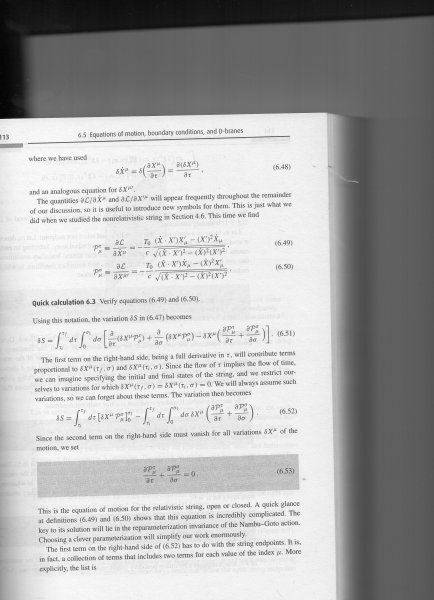# Unraveling the Mysteries of Relativistic Strings

• moriheru
In summary, the author is asking how to derive the P's for the relativistic string equation. The Nambu Goto Lagrangian he has derived is -\frac{T_0}{c} \sqrt{-det\gamma}=-\frac{T_0}{c} \sqrt{(\dot{X} X')(\dot{X}^2-\dot{X}^2X')^2} where the prefactor has been forgotten. For the other derivatives, the author says that if you have some problems with these inner products, you can use the orthonormality relation to solve for them.f

#### moriheru

My question concerns motion of relativistic Strings, as the reader with a great a mount of deductive skills can deduce :).The question is: How can I derive the P's. More acuratly speaking why is the numerator how it is.
I am referring to Zwiebach chapter 6.
Thanks for any clarifications and sorry about the zoom.

#### Attachments

Please type your question in, using LaTex if you need to express a formula. That's better than asking us to try and figure out what is in a tiny blurry picture.

Take the Lagrangian you have, and find its derivative...
The Nambu Goto lagrangian he has derived is : $L = - \frac{T_0}{c} \sqrt{ - det \gamma } = - \frac{T_0}{c} \sqrt{ ( \dot{X} X' )^2 - \dot{X}^2 X'^2 }$
Forgeting the prefactor:
$\frac{\partial L}{\partial \dot{X}^\rho} \propto \frac{2 ( \dot{X} X' ) X'_\rho - 2 X'^2 \dot{X}_\rho}{2 \sqrt{ ( \dot{X} X' )^2 - \dot{X}^2 X'^2 } }$
Similarily for the other...
If you have some problems with these derivatives of inner products:
$\frac{\partial }{\partial \dot{X}^\rho} ( \dot{X}^\mu X'_\mu )^2 =\frac{\partial }{\partial \dot{X}^\rho} \dot{X}^\mu X'_\mu \dot{X}^\nu X'_\nu = \delta_{\rho}^{\mu} X'_\mu \dot{X}^\nu X'_\nu + \dot{X}^\mu X'_\mu \delta_{\rho}^{\nu} X'_\nu = X'_\rho \dot{X}^\nu X'_\nu + \dot{X}^\mu X'_\mu X'_\rho =2 (\dot{X}^\nu X'_\nu ) X'_\rho$

The only thing you use is the orthonormality relation $\frac{\partial x^a}{\partial x^b}= \delta_b^a$

Last edited:
•moriheru
Take the Lagrangian you have, and find its derivative...
The Nambu Goto lagrangian he has derived is : $L = - \frac{T_0}{c} \sqrt{ - det \gamma } = - \frac{T_0}{c} \sqrt{ ( \dot{X} X' )^2 - \dot{X}^2 X'^2 }$
Forgeting the prefactor:
$\frac{\partial L}{\partial \dot{X}^\rho} \propto \frac{2 ( \dot{X} X' ) X'_\rho - 2 X'^2 \dot{X}_\rho}{2 \sqrt{ ( \dot{X} X' )^2 - \dot{X}^2 X'^2 } }$
Similarily for the other...
If you have some problems with these derivatives of inner products:
$\frac{\partial }{\partial \dot{X}^\rho} ( \dot{X}^\mu X'_\mu )^2 =\frac{\partial }{\partial \dot{X}^\rho} \dot{X}^\mu X'_\mu \dot{X}^\nu X'_\nu = \delta_{\rho}^{\mu} X'_\mu \dot{X}^\nu X'_\nu + \dot{X}^\mu X'_\mu \delta_{\rho}^{\nu} X'_\nu = X'_\rho \dot{X}^\nu X'_\nu + \dot{X}^\mu X'_\mu X'_\rho =2 (\dot{X}^\nu X'_\nu ) X'_\rho$

The only thing you use is the orthonormality relation $\frac{\partial x^a}{\partial x^b}= \delta_b^a$

Thanks! A like is yours.

Take the Lagrangian you have, and find its derivative...
The Nambu Goto lagrangian he has derived is : $L = - \frac{T_0}{c} \sqrt{ - det \gamma } = - \frac{T_0}{c} \sqrt{ ( \dot{X} X' )^2 - \dot{X}^2 X'^2 }$
Forgeting the prefactor:
$\frac{\partial L}{\partial \dot{X}^\rho} \propto \frac{2 ( \dot{X} X' ) X'_\rho - 2 X'^2 \dot{X}_\rho}{2 \sqrt{ ( \dot{X} X' )^2 - \dot{X}^2 X'^2 } }$
Similarily for the other...
If you have some problems with these derivatives of inner products:
$\frac{\partial }{\partial \dot{X}^\rho} ( \dot{X}^\mu X'_\mu )^2 =\frac{\partial }{\partial \dot{X}^\rho} \dot{X}^\mu X'_\mu \dot{X}^\nu X'_\nu = \delta_{\rho}^{\mu} X'_\mu \dot{X}^\nu X'_\nu + \dot{X}^\mu X'_\mu \delta_{\rho}^{\nu} X'_\nu = X'_\rho \dot{X}^\nu X'_\nu + \dot{X}^\mu X'_\mu X'_\rho =2 (\dot{X}^\nu X'_\nu ) X'_\rho$

The only thing you use is the orthonormality relation $\frac{\partial x^a}{\partial x^b}= \delta_b^a$

But why would one have a inner product?

What do you mean by why you have inner products? they are there when you wrote:

$\gamma = \begin{pmatrix} \dot{X} \cdot \dot{X} & \dot{X} \cdot X' \\ X' \cdot \dot{X} & X' \cdot X' \end{pmatrix}$
The $\cdot$ denotes inner product, and in this case the Lorentzian product $x \cdot y = \eta_{\mu \nu} x^\mu y^\nu$...
Now why is that the case? because you have already derived the induced metric:
$\gamma_{ab} = \eta_{\mu \nu} \partial_a X^\mu \partial_b X^\nu \equiv \partial_a X \cdot \partial_b X$

Or if you didn't ask exactly that, then remember:
$\frac{d}{dx} f(g(x)) = \frac{d}{dg}f(g) \frac{dg(x)}{dx}$

So the $\sqrt{...}$ will give the $\frac{1}{2\sqrt{...}}$
and you still have to take the derivatives of $(...)$ wrt your initial derivative.

•moriheru
Thanks fine from here!